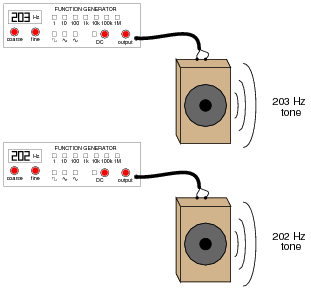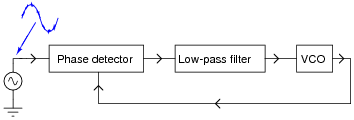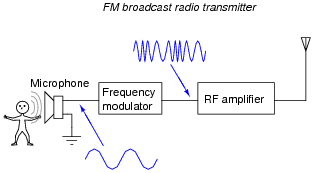Phase-Locked Loops

• Question 1

This is a schematic for a simple VCO:The oscillator is of the RC “phase shift” design. Explain how this circuit works. Why does the output frequency vary as the control voltage varies? Does the output frequency increase or decrease as the control voltage input receives a more positive voltage?

Hint: the JFETs in this circuit are not functioning as amplifiers!

• Question 2

If you have ever heard the sound of a dual-engine airplane flying overhead, you probably noticed an unusual “beat” pattern to the engines’ tone. The same phenomenon happens when you play the output of two audio signal generators through speakers, with the signal generators set to very similar (but not identical!) frequencies:Explain how this phenomenon could be used by a technician to adjust two audio frequency sources to the same frequency, without the aid of any expensive test equipment such as a frequency counter or an oscilloscope.

• Question 3

What is a VCO?

• Question 4

Suppose a technician connected a potentiometer to the input of a VCO, to act as a manually-variable voltage source. Then, the technician compares the output of the VCO with an external signal source that slowly changes frequency, adjusting the potentiometer to keep the two frequencies equal:If a voltmeter were connected between the potentiometer wiper and ground, what would its indication represent (with regard to the external audio signal)?

Explain where feedback occurs in this system, and how the system may be automated so as to not require the continual attention of a technician.

• Question 5

A block diagram of a phase-locked loop circuit looks like this:Determine what type of electronic signals would be seen at points A and B for the following input conditions:

Input = sine wave, steady frequency
Input = sine wave, increasing frequency
Input = sine wave, decreasing frequency
Input = sine wave, frequency increases and decreases regularly
• Question 6

Suppose that a “noisy” AC signal of otherwise constant frequency is connected to the input of a phase-locked loop circuit:Characterize the output waveform generated by the VCO. Will it be “noisy” as well? Why or why not?

• Question 7

Define the following terms, as they relate to phase-locked loop circuits:

Center frequency
Lock range
Capture range
Lock-up time
• Question 8

“FM” means “frequency modulation,” and it refers to the encoding of a waveform by changing (modulating) the frequency of another. This is the technology used in broadcast FM radio transmission:This is how FM radio communication works: by modulating the frequency of a radio-frequency (RF) signal according to the amplitude of the voltage signal produced by a microphone.

Explain how a phase-locked loop circuit could be used to “demodulate” the output of an FM radio station, so as to extract the broadcaster’s audio signal from the RF waveform.